# Retirement date calculator in Excel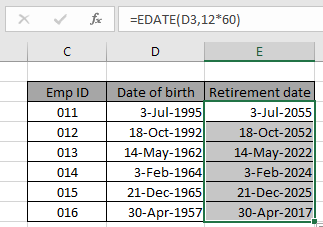In this article, we will learn how to calculate retirement date from given date of birth in Excel.

For this article we will be required to use the EDATE function. Now we will make a formula out of the function. Here we are given some data values in a range. We need to get the last working day for a person. We assume 60 years of working age for general purposes. You can customize the age as per requirement

Generic formula:-

= EDATE ( birth_date , 12*60 )

Birth_date : date of birth value

12*60 : total months in 60 years

Example:

All of these might be confusing to understand. So, let's test this formula via running it on the example shown below.

Here we have a list of dates of birth with Emp ID.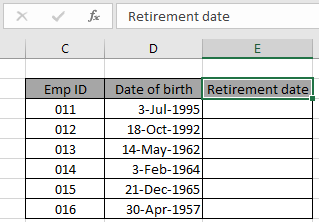Here we need to find the retirement date from the given date of birth values.

Use the formula in E3 cell:

= EDATE ( B3 , 12*60 )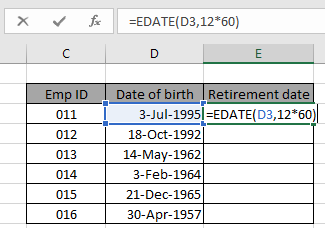Here is the date value that was given as cell reference. Press Enter to get the retirement date.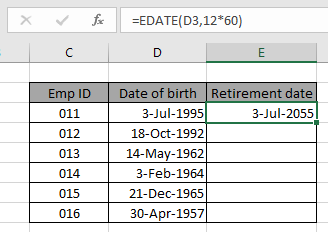As you can see the retirement date for the 011 Emp ID comes out to be July 3rd, 2055. Now copy the formula in other cells using the drag down option or using the shortcut key Ctrl + D as shown below.As you can see we obtained all employees' retirement age using the Excel EDATE function. Generally the returned date value is not in the required date format. Use the format cell option or Ctrl + 1 as shown in the below snapshot.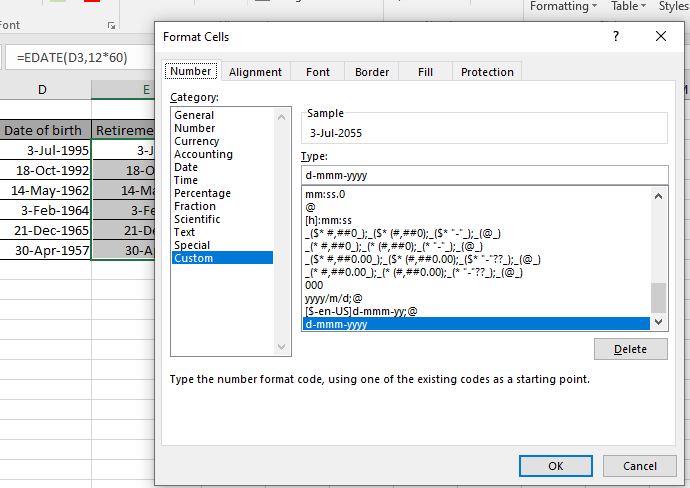Here are some observational notes shown below.

Notes:

1. The formula only works with numbers.
2. Do not use the data value directly in the formula. Use the DATE function or use cell reference as explained in the above example.

Hope this article about how to get the Retirement date calculator in Excel is explanatory. Find more articles on date & time formulas here.

If you liked our blogs, share it with your friends on Facebook. And also you can follow us on Twitter and Facebook. We would love to hear from you, do let us know how we can improve, complement or innovate our work and make it better for you. Write us at info@exceltip.com

Related Articles

How to use the EDATE function in Excel

Calculate age from date of birth

Calculate Weighted Average

How to Group Data By Age Range in Excel

Get day name from Date in Excel

Get Month name from Date in Excel

How to use wildcards in excel

Popular Articles

50 Excel Shortcut to Increase Your Productivity

Edit a dropdown list

Absolute reference in Excel

If with conditional formatting

If with wildcards

Vlookup by date

Terms and Conditions of use

The applications/code on this site are distributed as is and without warranties or liability. In no event shall the owner of the copyrights, or the authors of the applications/code be liable for any loss of profit, any problems or any damage resulting from the use or evaluation of the applications/code.